Latest Banking jobs   »   Arithmetic-2 Twisted One Quantitative Aptitude Quiz...

# Arithmetic-2 Twisted One Quantitative Aptitude Quiz for All Banking Exams- 21th May

Q1. A shopkeeper sells a product after allowing two successive discounts of 10% and 20% on it. Find the profit percent if the profit is 30% of the price by which the product is marked up?
(a) 30%
(b) 17.5%
(c) 25%
(d) 15%
(e) 20%

Q2. 45% of first number is equal to 60% of second number. If average of both the numbers is 10 less than the first number, then find 80% of second number?
(a) 64
(b) 40
(c) 48
(d) 56
(e) 80

Q3. Arun invested Rs. 10,000 for three years at CI at the rate of 20% per annum. If in 1st and 3rd year interest is calculated annually and in 2nd year it was calculated half-yearly, then find the total interest received by Arun in three years?
(a) Rs 7554
(b) Rs 7424
(c) Rs 7868
(d) Rs 7262
(e) Rs 7343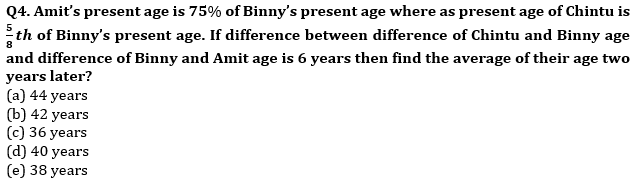Q5. Speed of boat in still water is 37.5% less than the speed of the boat in downstream and boat covers 30 km in upstream in 5 hours, then find time taken by boat to cover 84 km in downstream?
(a) 3.5 hr
(b) 3 hr
(c) 4.5 hr
(d) 4 hr
(e) 5 hr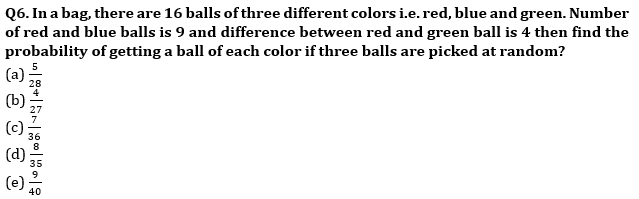Q7. Cost price of article A is Rs 600 more than that of B and selling price of A is Rs 1200 more than that of B. If difference between profit earned on selling these two articles is 13⅓% of the cost price of A then find profit earned on B if profit % earned on A is 33⅓%?
(a) Rs 800
(b) Rs 900
(c) Rs 840
(d) Rs 960
(e) Rs 1000

Q8. In 100 lit mixture of milk and water, milk is 68 lit more than that of water. When ‘a’ lit of milk is taken out and (a+15) lit of water is added, milk becomes 50% more than that of water. Find value of ‘a’?
(a) 20
(b) 15
(c) 18
(d) 12
(e) 16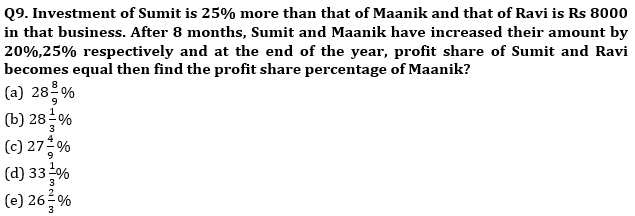Q10. Area of rectangle having length and breadth in the ratio of 4: 3 is 432 cm2. A square whose side is equal to diagonal of rectangle then find ratio of numerical value of perimeter to the area of square?
(a) 7:55
(b) 4:35
(c) None of these
(d) 1:8
(e) 2:15

Q11. Sahil invested a sum of Rs. 4000 at 10% compound interest compounding annually while Sanjay invested 50% more than Sahil at 20% simple interest. Find the ratio of interest received by Sahil & Sanjay in second year.
(a) 11 : 60
(b) 7 : 20
(c) 7 : 10
(d) 11 : 20
(e) 11 : 30

Q12. An urn contains 4 red, 5 green, 6 blue and some yellow balls. If two balls are drawn at random, the probability of getting at least one yellow ball is 17/38. find the yellow balls in the urn.
(a) 4
(b) 5
(c) 6
(d) 10
(e) 15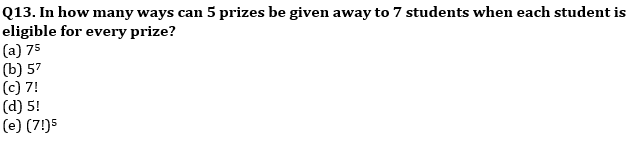Q14. The salary of Sumit is Rs. 50000 per month from which he spends 20% on rent, 10% of remaining on bills, 20% of further remaining on miscellaneous expenses. From the remaining amount, he gives some money to his wife and rest he invests in mutual funds in the ratio of 5 : 4. Amount spent on rent is how much less/more than amount invested in mutual funds.
(a) 2800 Rs.
(b) 2400 Rs.
(c) 2600 Rs.
(d) 3200 Rs.
(e) 3000 Rs.

Q15. A train engine can run at 56 kmph without any wagon. Decrease in speed of engine is directly proportional to square root of number of wagons attached. Speed becomes 40 kmph on attaching 4 wagons. Find maximum no. of wagons which engine can carry.
(a) 9
(b) 13
(c) 14
(d) 48
(e) 49

Practice More Questions of Quantitative Aptitude for Competitive Exams:

Solutions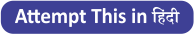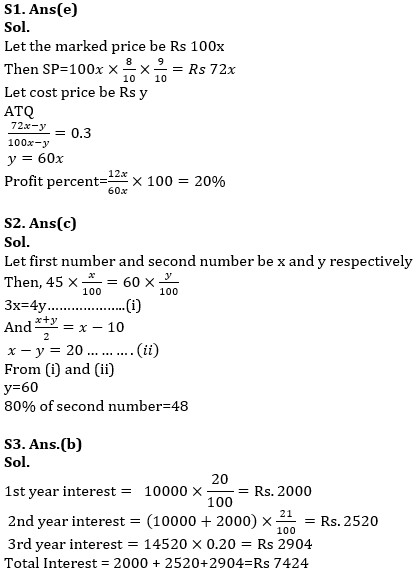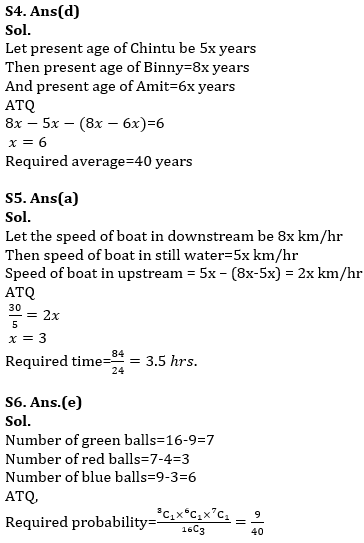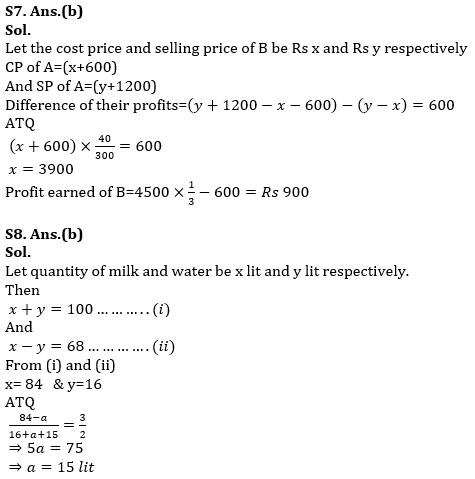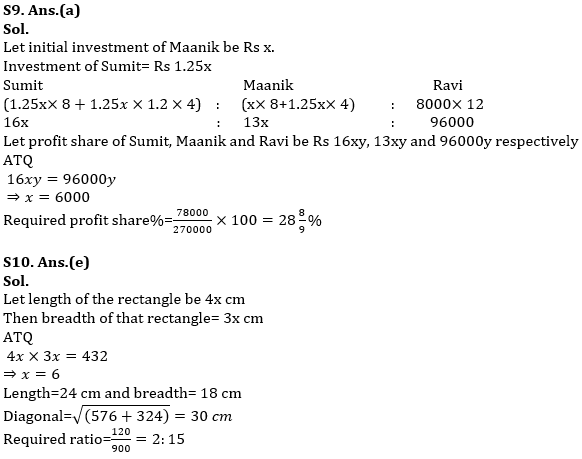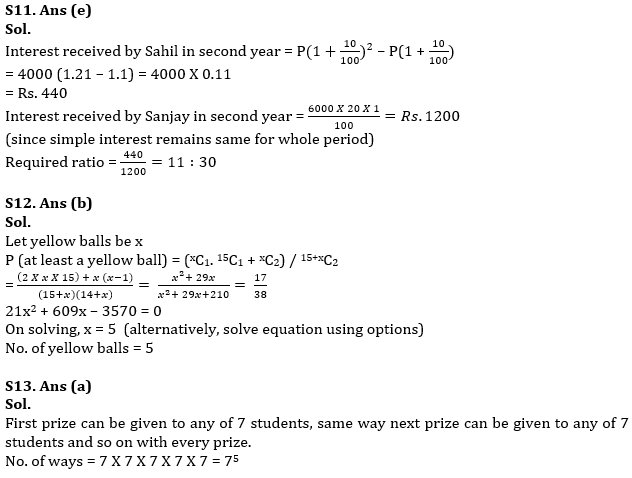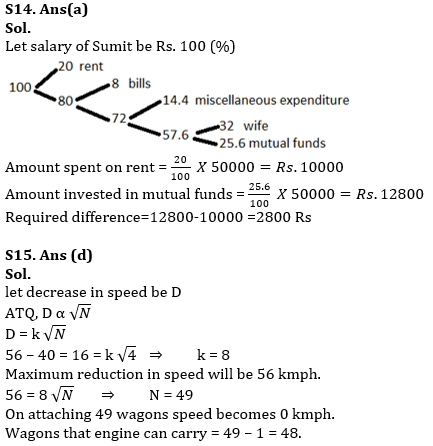#### Congratulations!Incorrect details? Fill the form again here

•Reasoning Ability Quiz For IBPS RRB PO C...
•Quantitative Aptitude Quiz For IBPS RRB ...
•Important Current Affairs Quiz for Bank ...
•Reasoning Ability Quiz For IBPS Clerk Pr...
•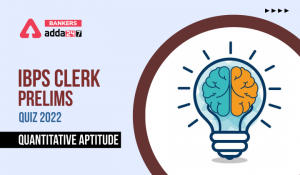Quantitative Aptitude Quiz For IBPS Cler...
•English Quizzes For IBPS Clerk Prelims 2...